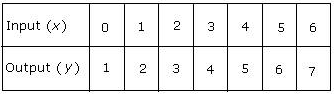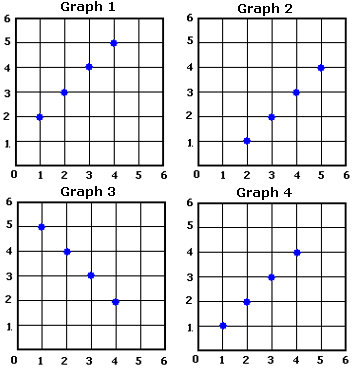## Definition Of Range Of A Function

The set of all output values or the y-values of a function or a relation is called the range of the function or the relation.

### Examples of Range of a Function

The table shows some of the input and the corresponding output values of the function y = x + 1.The range of the function is the set of all real numbers {. . . 1, 2, 3, 4, 5, 6, 7, . . . .}

### Solved Example on Range of a Function

#### Ques: x = 1, 2, 3, 4 are some of the input values of the function y = 6 - x. Find the range of the function for the given input values and then plot the values on a coordinate plane as ordered pairs.A. Graph 1
B. Graph 2
C. Graph 3
D. Graph 4

#### Solution:

Step 1: The range of the function y = 6 - x, varies as the value of x changes.
Step 2: The output value y for x = 1 is: y = 6 - 1 = 5. [Substitute 1 for x and add.]
Step 3: The output value y for x = 2 is: y = 6 - 2 = 4. [Substitute 2 for x and add.]
Step 4: The output value y for x = 3 is: y = 6 - 3 = 3. [Substitute 3 for x and add.]
Step 5: The output value y for x = 4 is: y = 6 - 4 = 2. [Substitute 4 for x and add.]
Step 6: The ordered pairs are (1, 5), (2, 4), (3, 3), (4, 2).
Step 7: Plot the points on a coordinate plane.Step 8: So, Graph 3 is the graph that has the range of the function for the given input values plotted correctly.